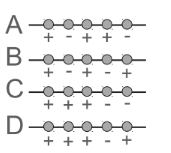# Problem: Five charges are equally spaced along the x axis. Each charge has the same magnitude e, but some of the charges are +e and some are −e. Four different configurations of charge are labelled A, B, C, D in the figure. Rank the magnitude of the force on the middle charge for the different configurations with largest first and smallest last. (1) C,D,A,B (2) C,A,D,B (3) D,C,A,B (4) A,C,D,B (5) C,B,D,A

###### FREE Expert Solution
79% (148 ratings)
###### Problem Details

Five charges are equally spaced along the x axis. Each charge has the same magnitude e, but some of the charges are +e and some are −e. Four different configurations of charge are labelled A, B, C, D in the figure. Rank the magnitude of the force on the middle charge for the different configurations with largest first and smallest last.

(1) C,D,A,B

(2) C,A,D,B

(3) D,C,A,B

(4) A,C,D,B

(5) C,B,D,A